Next: Polar Coordinates Up: Planetary Motion Previous: Newtonian Gravity

Conservation Laws

Now gravity is a conservative force. Hence, the gravitational force (210) can be written (see Section 2.5)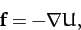(213)

where the potential energy,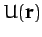, of our planet in the Sun's gravitational field takes the form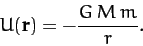(214)

It follows that the total energy of our planet is a conserved quantity--see Section 2.5. In other words,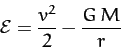(215)

is constant in time. Here,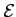is actually the planet's total energy per unit mass, and.

Gravity is also a central force. Hence, the angular momentum of our planet is a conserved quantity--see Section 2.6. In other words,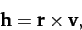(216)

which is actually the planet's angular momentum per unit mass, is constant in time. Taking the scalar product of the above equation with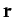, we obtain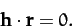(217)

This is the equation of a plane which passes through the origin, and whose normal is parallel to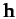. Sinceis a constant vector, it always points in the same direction. We, therefore, conclude that the motion of our planet is two-dimensional in nature: i.e., it is confined to some fixed plane which passes through the origin. Without loss of generality, we can let this plane coincide with the-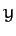plane.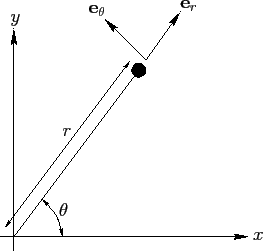Next: Polar Coordinates Up: Planetary Motion Previous: Newtonian Gravity
Richard Fitzpatrick 2011-03-31